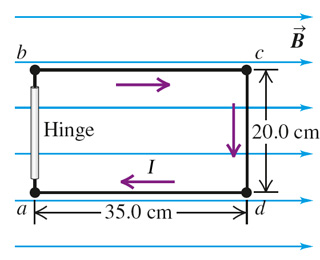# Problem: The 20.0 cm by 35.0 cm rectangular circuit shown in the figure is hinged along side ab. It carries a clockwise I = 5.35 A current and is located in a uniform 1.45 T magnetic field oriented perpendicular to two of its sides, as shown.a) Which force exerts a torque about the hinge ab?b) Calculate only the force that exert the torque mentioned in part A.c) Use your results from part B to calculate the torque that the magnetic field exerts on the circuit about the hinge axis ab.

###### FREE Expert Solution

Magnetic force on a current-carrying conductor:

$\overline{){{\mathbf{F}}}_{{\mathbf{B}}}{\mathbf{=}}{\mathbf{B}}{\mathbf{i}}{\mathbf{Lsin\theta }}}$

Torque:

$\overline{){\mathbit{\tau }}{\mathbf{=}}{\mathbit{r}}{\mathbit{F}}}$

(a)

Side ab has no force because the current i is zero.

For side bc, magnetic field and current are parallel (θ = 0°). sin 0 = 0. The force is zero.

94% (295 ratings)###### Problem Details

The 20.0 cm by 35.0 cm rectangular circuit shown in the figure is hinged along side ab. It carries a clockwise I = 5.35 A current and is located in a uniform 1.45 T magnetic field oriented perpendicular to two of its sides, as shown.a) Which force exerts a torque about the hinge ab?

b) Calculate only the force that exert the torque mentioned in part A.

c) Use your results from part B to calculate the torque that the magnetic field exerts on the circuit about the hinge axis ab.